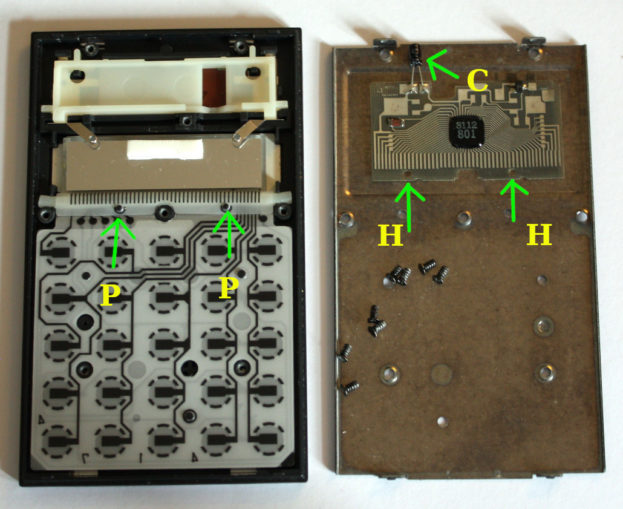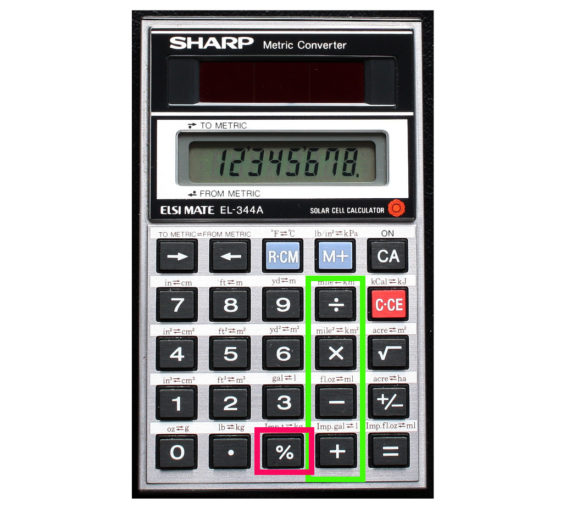# The Pocket Calculator### Introduction

Some of you may know that the Doc has been ill after falling off of a ladder. A very bad accident and I was lucky not to break anything (other than my confidence at climbing ladders). I’m okay and on the mend.

Whilst recuperating I thought about an old Sharp electronic calculator that I had cleaned up. An ‘ELSI MATE EL-344A’ from 1985. The solar panel contacts and the main display/keyboard contacts needed cleaning. The above photo shows the guts.

Note the two little posts (P) and the holes in the circuit board (H). These ensure that the gold/carbon contacts align correctly. Very clever. The circuit board is extremely thin, you have to be careful when removing or replacing it.

There’s a small capacitor (C). This “smooths out” any light transition (waving a hand over it – for example) from the solar panel. Think of it as a small battery.

It’s important that the eight screws (shown) are done up tight. All those connections have to be properly made.

### PercentagesAbove photo shows the four main operation keys +,-,$$\times$$,$$\div$$ and the % key

The percent key is a misunderstood operator on a simple pocket calculator.

We’re all familiar with taxation. The government nicks a percentage of what we work hard for (or buy) and gives it to… I’ll stop there.

Let’s focus on the four operator keys and the percent key of a basic pocket calculator. If you do this with, say, a home computer calculator app you might need to press the = equals key after the percent key.

Let’s start as simple as is possible. If I have a whole apple, I can say that I have 100% of an apple. Or one apple. Halve the apple and we have two 50% pieces of an apple or two 0.5 of an apple. As a decimal fraction:

$\text{Decimal Fraction} = {\text{Percent} \over 100}$

Therefore 50 $$\div$$ 100 = 0.5. If we simplify the fraction we get one half, or 1 $$\div$$ 2 = 0.5, as 50 divides into 100 twice.

This is how the calculator is working internally. For example, if we want to find out how much a £35 item is with VAT @20%?

$\text{Total Value} = {35 \times ({20 \over 100})} + 35 = £42$

On a pocket calculator this is very easy. Simply enter 35 + 20 % = £42.

### Subtraction And Division

Now this is where things get interesting and complicated. Let’s take our £42. Type into a calculator:

$\text{£42 – 20 % = £33.60}$

Hmm… Yes, it’s taken 20% off from £42, but that is not the original value of the product. It’s a correct answer, but suppose we want to know what the original value was?

It turns out that we can use the division key to “undo” our percentage. Example:

$\text{Original Value} = {42 \div ({120 \over 100})} = £35$

On a pocket calculator you’d simply enter 42 $$\div$$ 120 % = 35.

The smart ones amongst you may have noticed that the percentage has increased by 100%. Yes… Well. Stuff happens. In my teens I was “scaling 3D vector lines” (simple stuff). That’s when I got to thinking about ‘undoing’ percentages. It took awhile – I’m not that clever!

### Multiplication

If we take our apple analogy the mathematics is very simple. A whole apple is 100% and 50% is a half an apple.

$\text{Apple Size} = ({50 \over 100}) \times \text {One Apple} = 0.5$

On a pocket calculator simply enter 1 $$\times$$ 50 % = 0.5 or half of the original value. Remember that if we use our definition of percentage as a decimal fraction, above, 1 is the same as 100%.